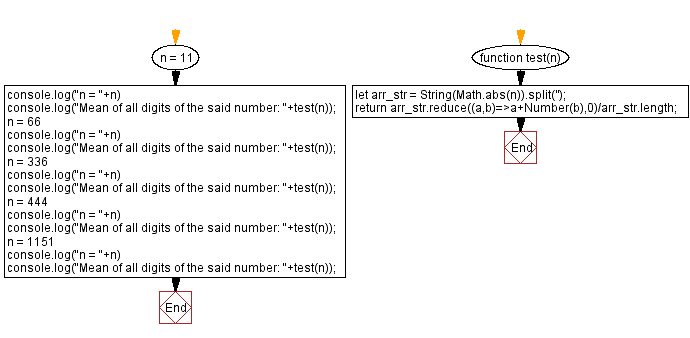# JavaScript: Mean of all digits of a number

## JavaScript Math: Exercise-82 with Solution

Write a JavaScript program to calculate the mean of all the digits of a given number.

What is a Mean?
In mathematics, the mean represents the simple average of two or more numbers. There are several methods available for computing the mean of a set of numbers, including the arithmetic mean method, which uses the sum of the numbers in the series, and the geometric mean method, which is the average of a set of products.

Test Data:
(11) -> 1
(66) -> 6
(336) -> 4
(444) -> 4
(1151) -> 2

Sample Solution:

HTML Code:

``````<!DOCTYPE html>
<html>
<meta charset="utf-8">
<title>JavaScript program to Mean of all digits of a number</title>
<body>

</body>
</html>
```
```

JavaScript Code:

``````function test(n) {
let arr_str = String(Math.abs(n)).split('');
return arr_str.reduce((a,b)=>a+Number(b),0)/arr_str.length;
}

n = 11
console.log("n = "+n)
console.log("Mean of all digits of the said number: "+test(n));
n = 66
console.log("n = "+n)
console.log("Mean of all digits of the said number: "+test(n));
n = 336
console.log("n = "+n)
console.log("Mean of all digits of the said number: "+test(n));
n = 444
console.log("n = "+n)
console.log("Mean of all digits of the said number: "+test(n));
n = 1151
console.log("n = "+n)
console.log("Mean of all digits of the said number: "+test(n));
```
```

Sample Output:

```n = 11
Mean of all digits of the said number: 1
n = 66
Mean of all digits of the said number: 6
n = 336
Mean of all digits of the said number: 4
n = 444
Mean of all digits of the said number: 4
n = 1151
Mean of all digits of the said number: 2
```

Flowchart:Live Demo:

See the Pen javascript-math-exercise-82 by w3resource (@w3resource) on CodePen.

Improve this sample solution and post your code through Disqus

What is the difficulty level of this exercise?

Test your Programming skills with w3resource's quiz.

﻿